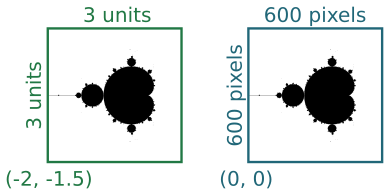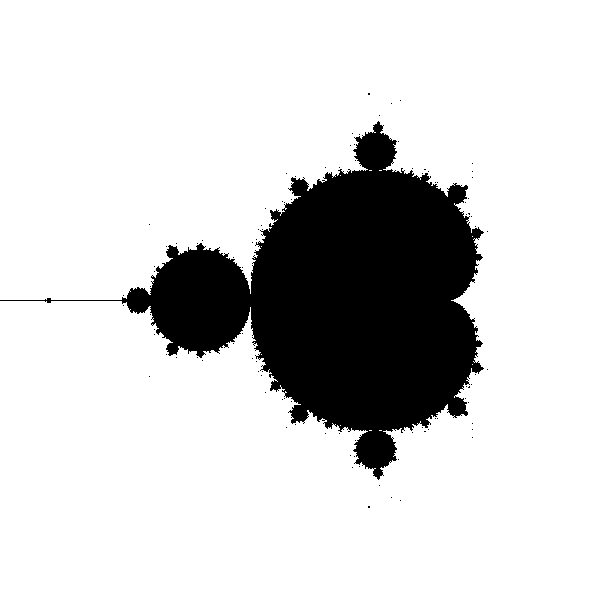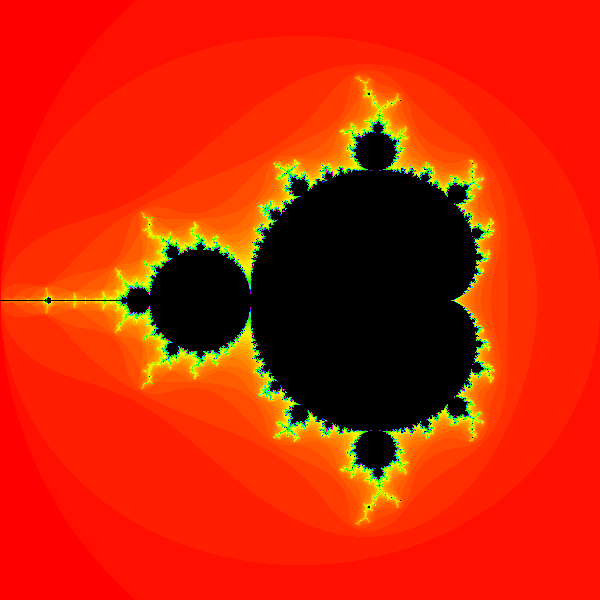# Mandelbrot set with generativepy

Martin McBride, 2020-10-28
Tags mandelbrot set
Categories generativepy generative art

generativepy has moved to the pythoninformer.com website, and this page might not be up to date. Please visit the new location.

The Mandelbrot set is a famous fractal that can be implemented fairly easily with generativepy.

The Mandelbrot set is an escape-time fractal. This article will explain how escape-time fractals work, using the Mandelbrot as an example.

## Escape-time fractals

The Mandelbrot set is based on an equation using complex numbers:

```znext = z*z + c
```

Where `z` is a complex variable, and `c` is a complex number constant. However, if you are not familiar with complex numbers we can rewrite this equation as a pair of equation in `x` and `y`:

```xnext = x*x - y*y + c1
ynext = 2*x*y + c2
```

Where `c1` and `c2` are two constant values.

Here are those equations implemented in a Python loop:

```def calc(c1, c2):
x = y = 0
for i in range(10):
x, y = x*x - y*y + c1, 2*x*y + c2
print(x, y)
```

We are interested in what happens as we call this function iteratively, with various values of `c1` and `c2`. Now if we try calling this function with `c1 = 0.2` and `c2 = 0.1`, the sequence of values looks like this:

```0.2 0.1
0.23 0.14
0.2333 0.16440000000000002
0.22740153000000002 0.17670904
0.22048537102861931 0.18036781212166242
0.21608125118807261 0.17953692795453008
0.21445759861565283 0.17758912805375543
0.21445416320109933 0.1761706758853121
0.21495448107239606 0.1755610697551134
0.2153837397195433 0.17547527729145027
```

After a few loops, the seem to stabilise - they converge. We could to run the loop many more times, the values would stay almost the same forever. If we try different values such as `c1 = 0.5` and `c2 = 1.0`, we get something very different:

```0.5 0.01
0.7499 0.02
1.06195001 0.039996000000000004
1.6261381437230003 0.09494750519992001
3.135310233727196 0.31879551971385567
10.228539678324857 2.0090457108504634
101.08675928277933 41.10920753800466
8529.065957891833 8311.20313340019
3668869.0894282013 141773599.43841496
-2.0086292897328776e+16 1040297553353152.1
```

The numbers get very big, very quickly - they diverge. If we ran the loop more times, the numbers would just get bigger and bigger.

## The Mandelbrot set

If we try this experiment with lots of different values for `c1` and `c2`, we will find that some values converge and some diverge. We could make a plot, representing the pair of values of `c1` and `c2`as the pixel at point `(c1, c2)`. We could make the pixel black if the converges, and white if it diverges. The set of all converging points are called the Mandelbrot set.

Since the equations are so simple, you might expect the shape created by the black pixels to also be simple - maybe a circle or something like that. But in fact the shape is incredibly intricate, the edge of the shape is so complex that you can zoom in on it forever and never run out of finer and finer detail.

To plot this shape, we will first tidy up our `calc` function:

```MAX_COUNT = 100

def calc(c1, c2):
x = y = 0
for i in range(MAX_COUNT):
x, y = x*x - y*y + c1, 2*x*y + c2
if x*x + y*y > 4:
return i
return -1
```

For a given pair of values, we iterate the function `MAX_COUNT` times. Each time through the loop it checks if the value `x*x + y*y` is greater than 4. That is the point of no return for the Mandelbrot equations, if that value is exceeded then it is never coming back, it is definitely going to diverge. In that case we return the loop count, which will be between 0 and `MAX_COUNT-1`.

However, if the exit condition hasn't happened within `MAX_COUNT` attempts, we will assume that it is never going to happen. The calculation has converged, the loop ends, so we return -1 to indicate that `(c1, c2)` is part of the Mandelbrot set.

## Plotting the image

To plot the image we will try lots of different combinations of `c1` and `c2`, and create a bitmap image with each pixel set to black if the point is inside teh set, or white otherwise.

It turns out that the interesting part of the Mandelbrot set is in a a region that is 3 units square, with its bottom corner at (-2, -1.5). In other words, x values between -2 and +1, and y values between -1.5 and +1.5. We would like to create an image 600 pixels square that represents this region:The two squares represent the same region, measured in different coordinate spaces We call the first set (the 3 by 3 square) our user space, and the second set (the 600 by 600 square) our pixel space.

We will use a `Scaler` object to convert between user space and pixel space. We create the scaler using the parameters above, then we can simple call:

```x, y = scaler.device_to_user(px, py)
```

to convert pixel coordinates to user coordinates.

## The drawing code

Here is the drawing code:

```from generativepy.bitmap import make_bitmap, Scaler
from generativepy.color import Color

BLACK = Color(0)
WHITE = Color(1)

def paint(image, pixel_width, pixel_height, frame_no, frame_count):
scaler = Scaler(pixel_width, pixel_height, width=3, startx=-2, starty=-1.5)

for px in range(pixel_width):
for py in range(pixel_height):
x, y = scaler.device_to_user(px, py)
count = calc(x, y)
col = BLACK if count < 0 else WHITE
image.putpixel((px, py), col.as_rgb_bytes())

make_bitmap('mandelbrot.png', paint, 600, 600)
```

First looking at the top level, we are using the `generativepy.bitmap.make_bitmap` function to create a bitmap image. This function accepts a `paint` function that does the actual drawing. The `paint` function is called with a PIL image object as its first parameter.

Within the paint function we create a `Scaler` object using the pixel width and height, and the user space width and offsets as mentioned above.

Now we have the main loop, which loops over every pixel in the output image. On each pass through the loop, `px` and `py` indicate the pixel coordinates of the current pixel. Here is the body of the main loop:

```x, y = scaler.device_to_user(px, py)
count = calc(x, y)
col = BLACK if count < 0 else WHITE
image.putpixel((px, py), col.as_rgb_bytes())
```

First we use the scaler to convert the pixel coordinates into use coordinates. `px` which has a range 0 to 599 is converted to a use range of -2.0 to +1.0. `py` which also has a range 0 to 599 is converted to a use range of 1.5 to -1.5.

We call `calc` to find out if the point is within the Mandelbrot set. Recall that the value is -1 for points that are in the set, and non-negative for points outside. We set `col` to black or white depending on the count.

Finally we use the PIL Image `putpixel` function to set the pixel `(px, py)` to the required colour.

generativepy colours are defined using float values 0.0 to 1.0 to define red, green and blue levels. Pillow uses integer value 0 to 255 instead. The `as_rgb_bytes` function converts a `Color` into a tuple of integers in the Pillow format.

Here is the image created:The black and white Mandelbrot is a little stark, and while it might be mathematically very interesting it lacks a little visual appeal.

There is something we can do fairly easily to rectify this. The `calc` function doesn't just return a true or false value. It actually returns:

• -1 if the point is inside the set.
• Otherwise, it returns a count of how many times the loop executes before the value of `x*x + y*y` exceeds 4 (the point of no return).

As you might expect, points that are a long way outside the boundary of the set tend to escape very quickly. Points that are very close to the boundary take longer to escape. So we can create a bit of extra interest in our image by setting the colour of the pixels outside the image according to the value returned by `calc`.

What we will do is create a colour table that maps a count value (in the range 0 to `MAX_COUNT-1`) onto a colour. Here is a function that creates a suitable table:

```def build_color_table(size):
table = [Color.of_hsl(i/size, 1, 0.5) for i in range(size)]
return table
```

This creates a table of HSL colours based on the count. The hue varies from 0.0 to 1.0 as the count increases from 0 to `size`. Th saturation stays at 1 and the lightness at 0.5. This creates a range of colours from red through yellow, green, and eventually blue.

In the main loop we simply change the line that sets the colour:

```col = BLACK if count < 0 else colors[count]
```

Here is the result:## The final code

Here is the final code for the colour Mandelbrot:

```from generativepy.bitmap import make_bitmap, Scaler
from generativepy.color import Color

MAX_COUNT = 100
BLACK = Color(0)

def calc(c1, c2):
x = y = 0
for i in range(MAX_COUNT):
x, y = x*x - y*y + c1, 2*x*y + c2
if x*x + y*y > 4:
return i
return -1

def build_color_table(size):
table = [Color.of_hsl(i/size, 1, 0.5) for i in range(size)]
return table

def paint(image, pixel_width, pixel_height, frame_no, frame_count):
scaler = Scaler(pixel_width, pixel_height, width=3, startx=-2, starty=-1.5)

colors = build_color_table(MAX_COUNT)

for px in range(pixel_width):
for py in range(pixel_height):
x, y = scaler.device_to_user(px, py)
count = calc(x, y)
col = BLACK if count < 0 else colors[count]
image.putpixel((px, py), col.as_rgb_bytes())

make_bitmap('mandelbrot-color.png', paint, 600, 600)
```

## Things to try

Here are a few things to try with the basic code.

Experiment with other colour schemes, you can use any scheme you like by modifying the `build_color_table` function. Remember that the outer areas of the image are mainly quite low values, whereas most of the higher values are concentrated closer to the edge of the set.

Create a super high resolution image by increasing the pixel dimensions you pass into `make_bitmap`. The scaling will automatically ensure that the same region of the image is visible. Be aware that every time you double the width and height the image will take 4 times longer to render.

Try zooming in on some other areas of the image by changing the `width`, `startx`, and `starty` you pass into the `Scaler`.